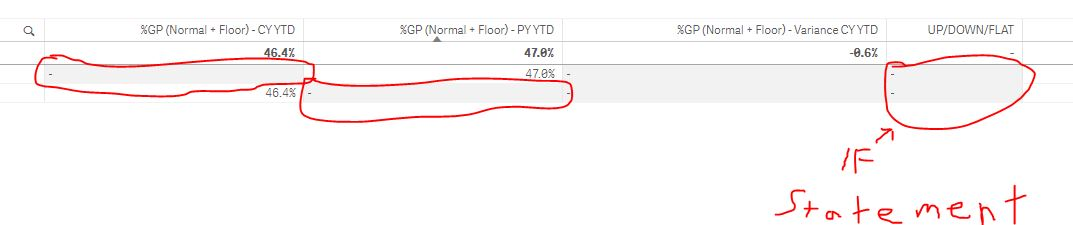# New to Qlik Sense

Discussion board where members can get started with Qlik Sense.

Announcements
Save \$300 - Register for QlikWorld (formerly Qonnections) by January 31st: Learn More
New Contributor

## What does a hyphen equal?

I have a report that returns a "hyphen" when there isn't any data in that timeframe.

My question would be, what does the Hyphen equal when I am composing a formula within an if statement?

10 Replies
Honored Contributor II

## Re: What does a hyphen equal?

They are nulls for that given value...

Ex:

If you have 5 dimensions and 1 measure, sometimes few of the rows will not show value for your measures but would show '-' as one of the dimensions in that table has valuePartner

## Re: What does a hyphen equal?

hyphen represents null values.

You can find it with functions like isnull(), len(trim()), or simply comparing like: if(field = "",...

Contributor II

## Re: What does a hyphen equal?

And, also confirm your field names are properly spelled in the condition.

New Contributor

## Re: What does a hyphen equal?

=if(num(sum(\$(Transaction_vSetYTD) \$(vProfit_True))/sum(\$(Transaction_vSetYTD)\$(vSales)),'#,##0.0%')='','No',Yes')

Contributor II

## Re: What does a hyphen equal?

what's the output that you're getting from the above expression? Is that a hyphen?

what does the below expressions give you

-> sum(\$(Transaction_vSetYTD) \$(vProfit_True))

-> sum(\$(Transaction_vSetYTD)\$(vSales))

If the above expressions are always giving you some values, then the output of your expression is always 'YES'.MVP

## Re: What does a hyphen equal?

Seems, You expression not returning Null values, Try this way?

=if(IsNull(num(sum(\$(Transaction_vSetYTD) \$(vProfit_True))/sum(\$(Transaction_vSetYTD)\$(vSales)),'#,##0.0%')),'No',Yes')

Before develop something, think If placed (The Right information | To the right people | At the Right time | In the Right place | With the Right context)
New Contributor

## Re: What does a hyphen equal?

I appreciate your suggestion, but that doesn't work.  it doesn't show a null value, just the hyphenNew Contributor

## Re: What does a hyphen equal?It doesn't always have a value.         First column is CY , second column is PY.     If no sales were in the CY or PY , it returns the  "Hyphen"

Contributor II

## Re: What does a hyphen equal?

what is your dimension here in this chart?